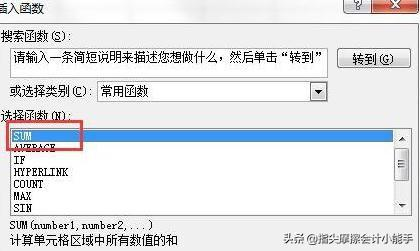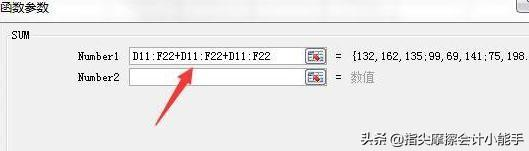• ## 常用求和公式和级数

万次阅读 多人点赞 2018-09-08 22:13:32
数列级数 ∑k=1∞k=12n(n+1)∑k=1∞k=12n(n+1)\sum\limits_{k=1}^{\infty}k=\dfrac{1}{2}n(n+1) ∑k=1nk2=16n(n+1)(2n+1)∑k=1nk2=16n(n+1)(2n+1)\sum\limits_{k=1}^{n}k^2=\dfrac{1}{6}n(n+1)(2n+1) ...
数列级数

∑k=1∞k=12n(n+1)

∑

k

=

1

∞

k

=

1

2

n

(

n

+

1

)

$\sum\limits_{k=1}^{\infty}k=\dfrac{1}{2}n(n+1)$

∑k=1nk2=16n(n+1)(2n+1)

∑

k

=

1

n

k

2

=

1

6

n

(

n

+

1

)

(

2

n

+

1

)

$\sum\limits_{k=1}^{n}k^2=\dfrac{1}{6}n(n+1)(2n+1)$

∑k=1nk3=14n2(n+1)2

∑

k

=

1

n

k

3

=

1

4

n

2

(

n

+

1

)

2

$\sum\limits_{k=1}^{n}k^3=\dfrac{1}{4}n^2(n+1)^2$

∑k=0∞xk=11−x

∑

k

=

0

∞

x

k

=

1

1

−

x

$\sum\limits_{k=0}^{\infty}x^k=\dfrac{1}{1-x}$，其中

|x|<1

|

x

|

<

1

$|x|<1$

∑k=0nxk=xn+1−1x−1

∑

k

=

0

n

x

k

=

x

n

+

1

−

1

x

−

1

$\sum\limits_{k=0}^nx^k=\dfrac{x^{n+1}-1}{x-1}$，其中

x≠1

x

≠

1

$x\neq 1$  函数项级数

∑n=0∞xnn!=ex,x∈(−∞,+∞)

∑

n

=

0

∞

x

n

n

!

=

e

x

,

x

∈

(

−

∞

,

+

∞

)

$\sum\limits_{n=0}^{\infty}\dfrac{x^n}{n!}=e^x,x\in (-\infty,+\infty)$

∑n=0∞(−1)n(2n+1)!x2n+1=sinx,x∈(−∞,+∞)

∑

n

=

0

∞

(

−

1

)

n

(

2

n

+

1

)

!

x

2

n

+

1

=

s

i

n

x

,

x

∈

(

−

∞

,

+

∞

)

$\sum\limits_{n=0}^{\infty}\dfrac{(-1)^n}{(2n+1)!}x^{2n+1}=sinx,x\in (-\infty,+\infty)$

∑n=1∞(−1)n(2n)!x2n=cosx,x∈(−∞,+∞)

∑

n

=

1

∞

(

−

1

)

n

(

2

n

)

!

x

2

n

=

c

o

s

x

,

x

∈

(

−

∞

,

+

∞

)

$\sum\limits_{n=1}^{\infty}\dfrac{(-1)^n}{(2n)!}x^{2n}=cosx,x\in (-\infty,+\infty)$
展开全文• ## 常用数列求和公式

千次阅读 2019-12-08 17:43:48
斐波那契数列 形如数列：1, 1, 2, 3, 5, 8, 13, 21, 34, 55, 89, 144, ... 如果设F(n）为该数列的第n项（n∈N*），那么这句话可以写成如下形式: F(n)=F(n-1)+F(n-2) 通项公式: ...则其前n项求和公式...
斐波那契数列
形如数列：1, 1, 2, 3, 5, 8, 13, 21, 34, 55, 89, 144, ...
如果设F(n）为该数列的第n项（n∈N*），那么这句话可以写成如下形式:
F(n)=F(n-1)+F(n-2)
通项公式:求和公式:
Sn=2An+A(n-1) - 1 = A(n+2) -1
等差数列：
形如数列：1, 2, 3, 4, 5, 6, ...
则其前n项求和公式如下：其中

a

1

=

第

一

项

，

a

n

=

最

后

一

项

，

d

=

差

值

a_1=第一项，a_n=最后一项 ， d=差值

等比数列：
数列形如：
1, 2, 4, 8, 16, 32, ...
通用公式：求和公式：以上就是在分析算法复杂度时，常用的数列求和公式和通用项描述。
另外算法的时间复杂度很多都用包含O(logN)这样的描述，但是却没有明确说logN的底数究竟是多少。这是因为n趋于无穷大时，logx(n)/logy(n)的极限可以发现，极限等于lny/lnx，也就是一个常数，所以从研究算法的角度log的底数不重要。
展开全文等差数列 斐波那契数列 等比数列
• http://blog.csdn.net/lewutian/article/details/4359766下面是常用的一些求和公式
http://blog.csdn.net/lewutian/article/details/4359766

下面是常用的一些求和公式：展开全文• 那Excel求和公式怎么用?今天，小编就和大家说一下Excel求和公式的使用方法和步骤。　步骤如下：　第1步：正常启动Excel，或双击自己的Excel文档。　Excel默认安装完毕，桌面是没有快捷相应的快捷键的，大家可打开...

有网友问到excel中能不能用函数求和，excel函数是很强大的功能，求和就不在话下了，在常用的Excel操作中，SUM求和是使用频率最高的一个函数。那Excel求和公式怎么用?今天，小编就和大家说一下Excel求和公式的使用方法和步骤。
步骤如下：
第1步：正常启动Excel，或双击自己的Excel文档。
Excel默认安装完毕，桌面是没有快捷相应的快捷键的，大家可打开Windows开始菜单中的所有程序下找到“Microsoft Office”，在此项下面却可找到‘Microsoft Excel 2010’此项，单击此项即可打开Excel软件了。
第2步：选中需要显示求和后所显示结果的单元格。
有些网友可能选中后，并不会象上图这样是实线，而是虚线，那么可能是别的地方还有公式或是复制什么了，这时大家需要按一下“ESC”键，然后再重新选择，正确就会如上图所示是实线。
第3步：选中需要显示的单元格之后，单击插入函数图标。
第4步：在打开的插入函数窗口中找到“SUM”求和函数并单击选中，然后单击“确定”按扭。当然如果直接双击SUM求和函数也可以。
第5步：在打开的SUM求和参数参数窗口中找到“Number1”后面的图标并单击。
第6步：单击鼠标左键不要松开，框选中自己需要求和函数求和的某一列或是某一区域，选择完毕之后按键盘中的回车键。
第7步： 再次回到Excel求和函数参数窗口，单击确定或按键盘中的回车键进行确认。第8步：确定之后，在本文第1步时所选中的单元格中就会使用Excel SUM求和函数自动计算出自己所选一区域中所有数字的和了。
补充：excel常用Ctrl组合快捷键
Ctrl+A全选
Ctrl+C复制
Ctrl+X剪切
Ctrl+V粘贴
Ctrl+D自动填充
Ctrl+B加粗
Ctrl+U下划线
Ctrl+I斜体
Ctrl+K超链接
Ctrl+F查找
Ctrl+H替换
Ctrl+L创建表
Ctrl+N新建工作簿
Ctrl+O打开文件
Ctrl+P打印
Ctrl+S保存
Ctrl+W关闭
Ctrl+Y撤销后重做
Ctrl+Z撤销关注小编获取更多知识了解

相关阅读：excel不常用的重要技巧
1.怎样快速打开设置单元格格式
我们经常在输入数值等的时候需要设置单元格格式，很多人都已经习惯了点击鼠标右键选项，但是我们可以使用快捷键CTRL加1快速打开，如果自己的手不在鼠标上非常方便。
当然，我们也可以用鼠标点击如下图所示位置快速打开设置单元格格式选项。
2.excel怎样设置小数位数
如果一行带小数的数字我们要快速转化为整数的话可以使用快捷键CTRL加SHIFT加1，设置起来速度非常快。
当然，我们经常不会只使用整数，小数位的缩进或者延伸我们可以选中要设置的单元格后使用点击下面两个选项进行设置。
3.关闭excel表的快捷方式
我们经常需要打开一个表格完成工作后需要关闭，可以使用快捷方式CTRL加W，使用习惯后比较方便，希望能够对大家有帮助。

展开全文excel保存快捷键
• 转自：http://www.pinlue.com/article/2020/12/2012/1011431576520.htmlexcel
• 下面是常用的一些求和公式：其中有部分求和公式在数学竞赛中是经常用到的！
• 编按：按条件求和，工作中很常见。如果是根据条件求单列数据之和，SUMIF函数即可解决，但如果是求多列数据呢？我们这里分享12种方法，各有各的特色。学习更多技巧，请收藏关注部落窝教育excel图文教程。先来看一下...
• ## 数列求和公式汇总

万次阅读 2017-08-06 18:51:07
常见公式 1+2+3+…+n=n(n+1)/2 ...{//等差数列求和公式 return (n+1)*n/2;//注意(n+1)/2*n这样不对 } q^1+q^2+q^3+…+q^n=q*(q^n-1)/(q-1) LL power(int a,int b){ LL ans=1,base=a; while(b&gt;0)...
• 等差数列求和公式1.公式法2.错位相减法3.求和公式4.分组法有一类数列，既不是等差数列，也不是等比数列，若将这类数列适当拆开，可分为几个等差、等比或常见的数列，然后分别求和，再将其合并即可.5.裂项相消法适用...
• ”别担心，今天老菜鸟将给大家分享三种用公式直接忽略错误值求和的方法，赶紧来看看吧！＊＊＊＊＊＊＊＊＊＊＊＊＊＊＊按照对应的订单号引用已有的收货金额，这种问题相信很多朋友都会处理，用VLOOKUP...
• ## 组合数有关的公式及常用求和

万次阅读 多人点赞 2017-08-16 13:06:09
• ## 数列求和常用公式

千次阅读 2007-04-20 09:59:00
1）1+2+3+......+n=n(n+1)÷2 2）1^2+2^2+3^2+......+n^2=n(n+1)(2n+1)÷6 3） 1^3+2^3+3^3+......+n^3=( 1+2+3+......+n)^2 =n^2*(n+1)^2÷4 4） 1*2+2*3+3*4+......+n(n+1) =n(n+1)(n+2)÷3 5） 1*2*3+2*3
• ## 等差数列求和公式

千次阅读 2018-10-08 11:09:22
等差数列是常见数列的一种，可以用AP表示，如果一个数列从第二项起，每一项与它的前一项的差等于同一个常数，这个数列就叫做等差数列，而这个常数叫做等差数列的公差，公差常用字母d表示。例如：1,3,5,7,9……（2n-1...
• 求和函数，是我们开始学习Execl时就学习到的技能，也是数据统计使用最频繁的函数，点击相应的命令或者利用sum函数来完成。其实，求和不仅仅只有命令或sum函数可以完成，还可以与快捷键、条件求和、相对绝对引用结合...
• 以下公式来源来源：https://blog.csdn.net/bigtiao097/article/details/77242624 Cnm=Cn−1m−1+Cn−1mC_n^m = C _{n-1}^{m-1}+C _{n-1}^{m}Cnm​=Cn−1m−1​+Cn−1m​ mCnm=nCn−1m−1mC_n^m = nC _{n-1}^{m-1}...算法
• 在excel中，我们经常用到求和计算，在该软件中，求和计算是可以自动进行的，那么Excel2003中的数据如何求和?如何用RANK排名次？...公式编辑完毕后，单击编辑栏左边的勾，或者按回车键执行计算命令。...
• https://www.cnblogs.com/shengwang/p/9836236.html
• 方法一、使用“+”加号求和这种方法是最简单也是最常用的一种方法，适合新手菜鸟朋友，直接在E3单元格输入“=”，然后光标点击需要...方法二、利用Excel中自带求和公式选中某行或者某列需要进行求和的数据后，点击【...
• 计算级数∑最常用的方法是数学归纳法。例如，我们来证明等差级数命题 n ∑ k 的值等于1/2*n(n+1) k=1 容易看出，当n=1时，这一结论是成立的，因此可以归纳假设对n成立，并证明对n+1成立。我们有 n+1 n ∑ k ...
• 等比数列公式： 通用公式： a1为第一项 n为所有的项数 q 等比数列的公比 am为第一项 n为所有的项数 q 等比数列的公比 求和公式 对数常用的公式 ...
• 这一节只是介绍了一些求和公式，本来并没有什么体会要写，但是后面有句话使我折服了：上述的公式很多教材是先简单地陈述了这个公式，然后用数学归纳法去证明，当然这样可以完美地证明出来，但是我们并不知道第一次...算法...